Home > INT3 > Chapter 7 > Lesson 7.2.2 > Problem7-69

7-69.
1.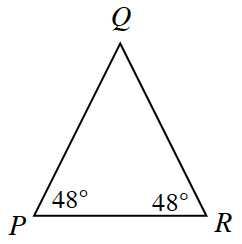Beatriz notices that two angles in ΔPQR, shown at right, have the same measure. Homework Help ✎

1. Based on this information, what statement can you make about the relationship between QP and QR ?

2. If QP = 5 mm, what is the length of PR ?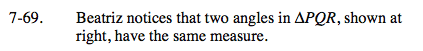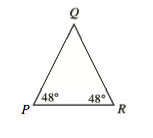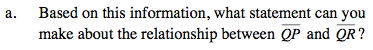The base angles have equal measure. What kind of triangle is this?

The stated sides must have equal length.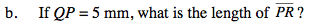Calculate the measure of the missing angle.

Can you use the Law of Sines to find your solution?

≈ 6.67 mm